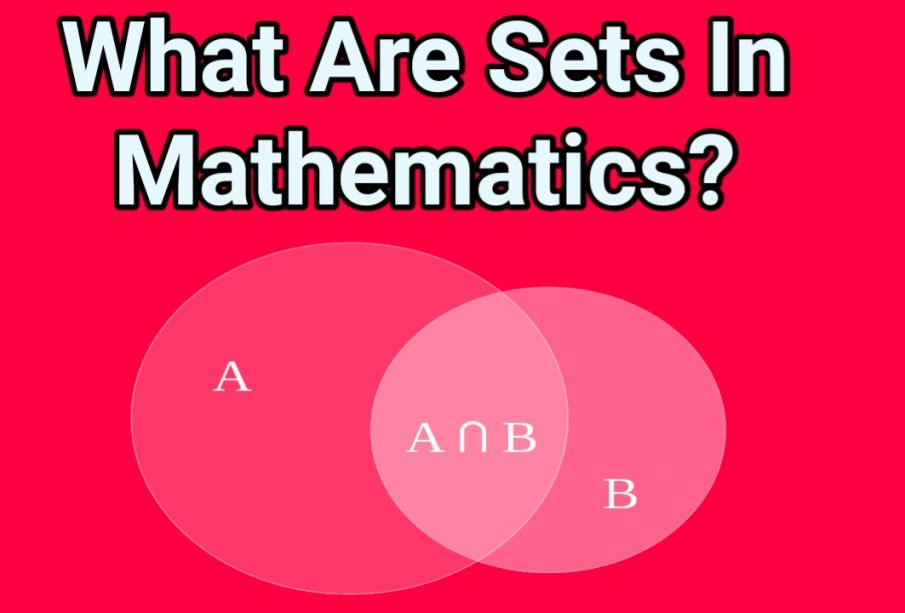Thursday, September 28

# What are the Types of Sets in Maths?

0
517In Maths, sets represent the collections of objects or collections of elements. Numbers, variables, alphabets, etc. could be the elements of the sets, depending on conditions. A set is denoted by curly brackets ‘{}’.

For example, if A is set of all vowels, then it is represented as:

A = {a, e, i, o, u}

This form of representation of the set is called Roster Form. If we have to represent the same set in Set Builder form, then it can be done as:

A = {x: x is all vowels in English alphabets}

In the sets’ theory, we will come across different types of sets as well, such as :

• Subset
• Finite set
• Infinite set
• Empty set
• Singleton set
• Equal set
• Equivalent set
• Power set
• Universal set

Let us learn them one by one.

Subset: A subset is a set derived from its parent set or original set. If set A has elements which are all also present in set B, then set A is the subsets of set B. It is denoted as A ⊆ B.

For example, A = {a,b}, then subset of A are {}, {a}, {b} and {a,b}.

Finite set: If a set has a finite number of elements, then it is called a finite set. For example,

Set A = {x: x is the name of months in a year}

Hence, there will be only twelve names.

Infinite set: A set which has an infinite number of elements, is known as an infinite set.

Example: Set A = {x: x is whole number}

Empty Set: A set which contains no elements in it, is called empty set or null set.

Singleton set: A set which has only one element is called a singleton set.

Example: Set A = {1}

Or Set A = {x: x is even prime number}, which is 2.

Equal Sets: When any two sets have exactly the same elements, then they are equal. The order of the elements does not matter.

Example: Set A = {12, 23, 45} and Set B = {45, 23, 12}

So, Set A is equal to Set B

Equivalent Sets: If any two sets have an equal number of elements, then they are said to be equivalent to each other.

Example: Set A = {1,2,5,6,7} and Set B = {a,b,c,d,e}

Since, both the sets A and B have elements equal to five, therefore they are equivalent.

Power Set: A power set is a set which consists of all the subsets, including the empty set and the parent set itself. If A is any set, then the power set is denoted by P(A).

Example: A = {1,5,7}, then power set P(A) will be;

P(A) = {}, {1}, {5}, {7}, {1,5}, {1,7}, {5,7}, {1,5,7}

Universal Set: A set which consists of all the sets is called a universal set. It is the base or home for all the sets, based on a particular context. It is usually denoted by the alphabet ‘U’.

For example, a set of integers is a universal set of all positive integers, negative integers and zero.

These were the primary types of sets which we learn in Maths. The operations of sets are majorly based on all these types.